## Example Questions

### Example Question #1 : How To Graph An Equation With A Number Line

If 0 < n < 1, then which of the following is the smallest?

n3 – 1

n2

1/n2

–1/n3

–1/n

–1/n3

Explanation:

First, it will help us to determine which of the answer choices are positive and which are negative.

Because n is positive, we know that n2 is positive, because any number squared is positive. Similarly, 1/n2 is also positive.

Let's look at the answer choice –1/n. This must be negative, because a negative number divided by a positive one will give us a negative number. Similarly, –1/n3 will also be negative.

The last choice is n3 – 1. We are told that 0 < n < 1. Because n is a positive value less than one, we know that 0 < n3 < n2 < n < 1. In other words, n3 will be a small positive value, but it will still be less than one. Thus, because n3 < 1, if we subtract 1 from both sides, we see that n3 – 1 < 0. Therefore, n3 - 1 is a negative value.

All negative numbers are less than positive numbers. Thus, we can eliminate n2 and 1/n2, which are both positive. We are left with –1/n, –1/n3, and n3 – 1.

Let us compare –1/n and –1/n3. First, let us assume that –1/n < –1/n3.

–1/n < –1/n3

Multiply both sides by n3. We don't need to switch the signs because n3 is positive.

–n< –1

Multiply both sides by –1.

n2 > 1.

We know that n2 will only be bigger than 1 when n > 1 or if n < –1. But we know that 0 < n < 1, so –1/n is not less than –1/n3. Therefore, –1/nmust be smaller.

Finally, let's compare –1/n3 and n3 – 1. Let us assume that –1/n3 < n3 – 1.

–1/n3 < n3 – 1

Multiply both sides by n3.

–1 < n6 – n3

n6 – n3 + 1 > 0. If this inequality is true, then it will be true that –1/n3 is the smallest number.

Here it will be helpful to try some values for n. Let's pick n = 1/2 and see what happens. It will help to use our calculator.

(1/2)6 – (1/2)3 + 1 = 0.891 > 0.

Therefore, we suspect that because n6 – n3 + 1 > 0, –1/nis indeed the smallest number. We can verify this by trying more values of n.

### Example Question #1 : How To Graph An Inequality With A Number Line

On a real number line, x1 = -4 and x2 = 14. What is the distance between these two points?

4

18

10

-18

18

Explanation:

The distance between two points is always positive. We calculate lx2 - x1l, which will give us the distance between the points.

|14- (-4)| = |14+4| = |18| = 18

### Example Question #3 : How To Graph An Inequality With A Number Line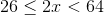Which of the following is a graph for the values ofdefined by the inequality stated above?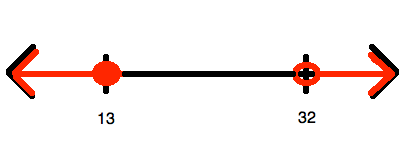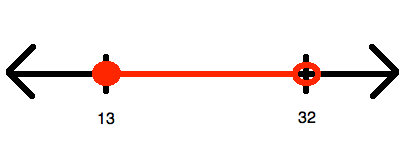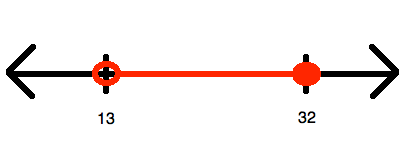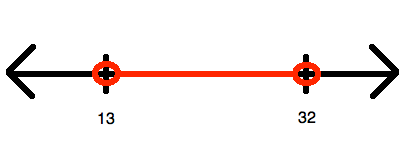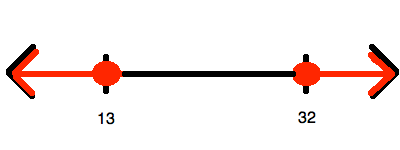Explanation:

To begin, you must simplify so that you "isolate", (i.e. at least eliminate any coefficients from it). To do this, divide all of the members of the inequality by: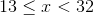Now, this inequality represents all of the numbers between 13 and 32.  However, it does include(hence, getting a closed circle for that value) and does not include(hence, getting an open circle for that value). Therefore, it looks like:### Example Question #4 : How To Graph An Inequality With A Number Line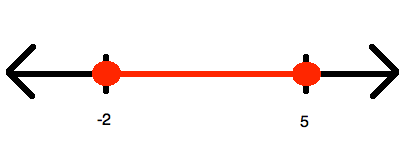Which of the following inequalities is represented by the number line shown above?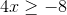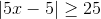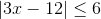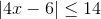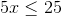Explanation:

Since the inequality represents one range of values between two end points (both of which are included, given the sign being "less than or equal"), you know that whatever you answer, it must be convertible to the form: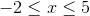Now, you know that it is impossible to get this out of the choices that have no absolute values involved in them. Therefore, the only options that make sense are the two having absolute values; however, here you should choose only the ones that have a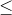, for only that will yield a range like this. Thus, we can try both of our options.

The wrong answer is simplified in this manner: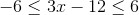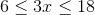And you can stop right here, for you know you will never havefor the left terminus.

The other option is simplified in this manner: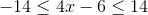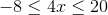This is just what you need!

### Example Question #5 : How To Graph An Inequality With A Number Line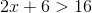Which of the following is a graph for the values ofdefined by the inequality stated above?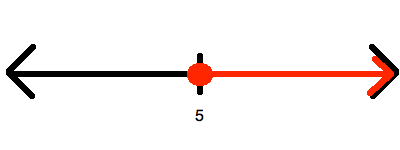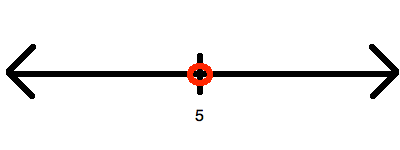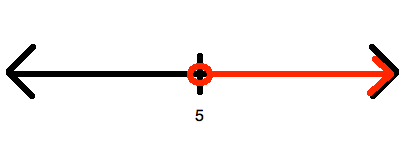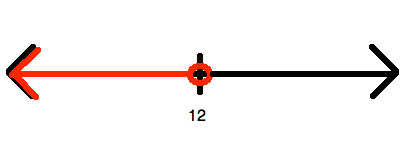Explanation:

Begin by solving for: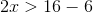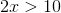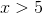Now, this is represented by drawing an open circle at 6 and graphing upward to infinity:### Example Question #1 : How To Find Value With A Number Line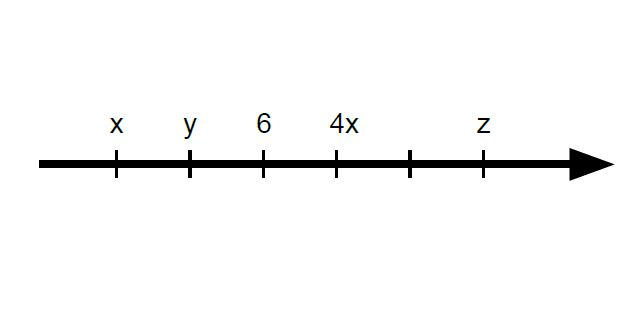If the tick marks are equally spaced on the number line above, what is the average (arithmetic mean) of x, y, and z?

5

7

6

4

8

6

Explanation:

First, we must find out by how much they are spaced by. It cannot be 1, since 4(4) = 16, which is too great of a step in the positive direction and exceeds the equal-spacing limit. 2 works perfectly, however, as 4(2) equals 8 and fits in line with the equal spacing.

Next, we can find the values of x and y since we are given a value of 6 for the third tick mark. As such, x (6 – 4) and y (6 – 2) are 2 and 4, respectively.

Finally, z is 4 steps away from y, and since each step has a value of 2, 2(4) = 8, plus the value that y is already at, 8 + 4 = 12 (or can simply count).

Finding the average of all 3 values, we get (2 + 4 + 12)/3 = 18/3 = 6.

### Example Question #2 : How To Find Value With A Number Line

How many numbers 1 to 250 inclusive are cubes of integers?Explanation:

The cubes of integers from 1 to 250 are 1, 8, 27,64,125,216.

### Example Question #3 : How To Find Value With A Number Line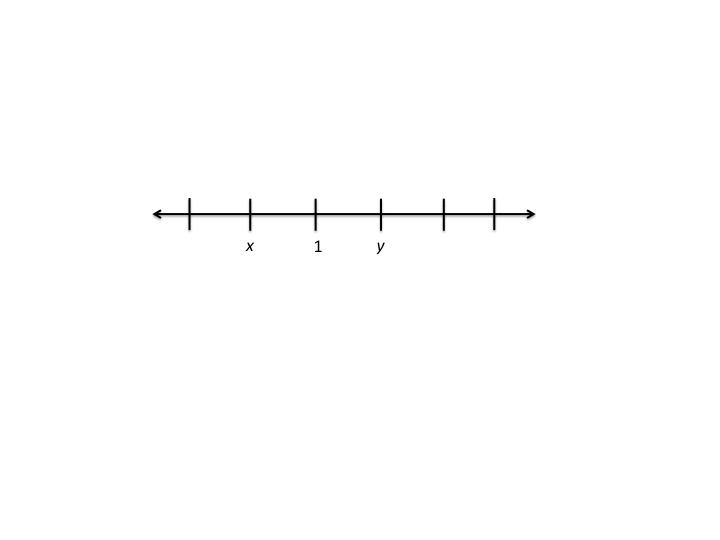On the number line above, the tick marks are equally spaced. Which of the following expressesin terms of?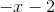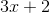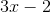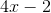Ifis betweenandon the number line, thenand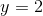.
Somust be correct because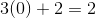.